2022: SklogWiki celebrates 15 years on-line

# Difference between revisions of "Stirling's approximation"

Stirling's approximation is named after the Scottish mathematician James Stirling (1692-1770).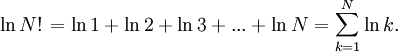$\left.\ln N!\right. = \ln 1 + \ln 2 + \ln 3 + ... + \ln N = \sum_{k=1}^N \ln k .$

using Euler-MacLaurin formula one has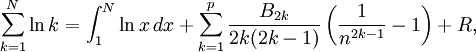$\sum_{k=1}^N \ln k=\int_1^N \ln x\,dx+\sum_{k=1}^p\frac{B_{2k}}{2k(2k-1)}\left(\frac{1}{n^{2k-1}}-1\right)+R ,$

where B1 = −1/2, B2 = 1/6, B3 = 0, B4 = −1/30, B5 = 0, B6 = 1/42, B7 = 0, B8 = −1/30, ... are the Bernoulli numbers, and R is an error term which is normally small for suitable values of p.

Then, for large N,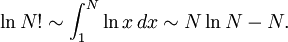$\ln N! \sim \int_1^N \ln x\,dx \sim N \ln N -N .$

after some further manipulation one arrives at (apparently Stirling's contribution was the prefactor of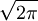$\sqrt{2 \pi}$)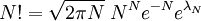$N! = \sqrt{2 \pi N} \; N^{N} e^{-N} e^{\lambda_N}$

where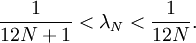$\frac{1}{12N+1} < \lambda_N < \frac{1}{12N}.$

For example:

 N N! (exact) N! (Stirling) Error (%) 2 2 1.91900435 4.22 3 6 5.83620959 2.81 4 24 23.5061751 2.10 5 120 118.019168 1.67 6 720 710.078185 1.40 7 5040 4980.39583 1.20 8 40320 39902.3955 1.05 9 362880 359536.873 0.93 10 3628800 3598695.62 0.84

When one is dealing with numbers of the order of the Avogadro constant (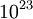$10^{23}$) this formula is essentially exact. In computer simulations the number of atoms or molecules (N) is invariably greater than 100; for N=100 the percentage error is approximately 0.083%.